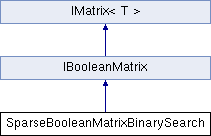MyMediaLite  3.01
SparseBooleanMatrixBinarySearch Class Reference

Sparse representation of a boolean matrix, using binary search (memory efficient) More...

Inheritance diagram for SparseBooleanMatrixBinarySearch:List of all members.

## Public Member Functions

IMatrix< bool > CreateMatrix (int x, int y)
Create a matrix with a given number of rows and columns.
IList< int > GetEntriesByColumn (int column_id)
IList< int > GetEntriesByRow (int row_id)
Get all true entries (column IDs) of a row.
void Grow (int num_rows, int num_cols)
Grows the matrix to the requested size, if necessary.
int NumEntriesByColumn (int column_id)
Get all the number of entries in a column.
int NumEntriesByRow (int row_id)
Get all the number of entries in a row.
int Overlap (IBooleanMatrix s)
Get the overlap of two matrices, i.e. the number of true entries where they agree.
IMatrix< bool > Transpose ()
Get the transpose of the matrix, i.e. a matrix where rows and columns are interchanged.

## Protected Attributes

internal IList< List< int > > row_list = new List<List<int>>()
internal representation of this data: list of rows

## Properties

virtual bool IsSymmetric` [get]`
True if the matrix is symmetric, false otherwise.
IList< int > NonEmptyColumnIDs` [get]`
The IDs of the non-empty columns in the matrix (the ones that contain at least one true entry)
IList< int > NonEmptyRowIDs` [get]`
The IDs of the non-empty rows in the matrix (the ones that contain at least one true entry)
IList< KeyValuePair< int,
IList< int > > >
NonEmptyRows` [get]`
The non-empty rows of the matrix (the ones that contain at least one true entry), with their IDs.
int NumberOfColumns` [get]`
The number of columns in the matrix.
int NumberOfEntries` [get]`
The number of (true) entries.
int NumberOfRows` [get]`
The number of rows in the matrix.
bool this[int x, int y]` [get, set]`
The value at (i,j)
ICollection< int > this[int x]` [get]`
Indexer to access the rows of the matrix.

## Detailed Description

Sparse representation of a boolean matrix, using binary search (memory efficient)

Fast row-wise access is possible. Indexes are zero-based.

## Member Function Documentation

 IMatrix CreateMatrix ( int num_rows, int num_columns ) ` [inline]`

Create a matrix with a given number of rows and columns.

Parameters:
 num_rows the number of rows num_columns the number of columns
Returns:
A matrix with num_rows rows and num_column columns

Implements IMatrix< T >.

 IList GetEntriesByColumn ( int column_id ) ` [inline]`

Takes O(N log(M)) worst-case time, where N is the number of rows and M is the number of columns.

Implements IBooleanMatrix.

 IList GetEntriesByRow ( int row_id ) ` [inline]`

Get all true entries (column IDs) of a row.

Parameters:
 row_id the row ID
Returns:
a list of column IDs

Implements IBooleanMatrix.

 void Grow ( int num_rows, int num_cols ) ` [inline]`

Grows the matrix to the requested size, if necessary.

The new entries are filled with zeros.

Parameters:
 num_rows the minimum number of rows num_cols the minimum number of columns

Implements IMatrix< T >.

 int NumEntriesByColumn ( int column_id ) ` [inline]`

Get all the number of entries in a column.

Parameters:
 column_id the column ID
Returns:
the number of entries in column column_id

Implements IBooleanMatrix.

 int NumEntriesByRow ( int row_id ) ` [inline]`

Get all the number of entries in a row.

Parameters:
 row_id the row ID
Returns:
the number of entries in row row_id

Implements IBooleanMatrix.

 int Overlap ( IBooleanMatrix s ) ` [inline]`

Get the overlap of two matrices, i.e. the number of true entries where they agree.

Parameters:
 s the IBooleanMatrix to compare to
Returns:
the number of entries that are true in both matrices

Implements IBooleanMatrix.

 IMatrix Transpose ( ) ` [inline]`

Get the transpose of the matrix, i.e. a matrix where rows and columns are interchanged.

Returns:
the transpose of the matrix

Implements IMatrix< T >.

## Member Data Documentation

 internal IList > row_list = new List>()` [protected]`

internal representation of this data: list of rows

## Property Documentation

 virtual bool IsSymmetric` [get]`

True if the matrix is symmetric, false otherwise.

True if the matrix is symmetric, false otherwise

Implements IMatrix< T >.

 IList NonEmptyColumnIDs` [get]`

The IDs of the non-empty columns in the matrix (the ones that contain at least one true entry)

Implements IBooleanMatrix.

 IList NonEmptyRowIDs` [get]`

The IDs of the non-empty rows in the matrix (the ones that contain at least one true entry)

Implements IBooleanMatrix.

 IList > > NonEmptyRows` [get]`

The non-empty rows of the matrix (the ones that contain at least one true entry), with their IDs.

The non-empty rows of the matrix (the ones that contain at least one true entry), with their IDs

 int NumberOfColumns` [get]`

The number of columns in the matrix.

The number of columns in the matrix

Implements IMatrix< T >.

 int NumberOfEntries` [get]`

The number of (true) entries.

The number of (true) entries

Implements IBooleanMatrix.

 int NumberOfRows` [get]`

The number of rows in the matrix.

The number of rows in the matrix

Implements IMatrix< T >.

 bool this[int x, int y]` [get, set]`

The value at (i,j)

The value at (i,j)

Parameters:
 x the row ID y the column ID

Implements IMatrix< T >.

 ICollection this[int x]` [get]`

Indexer to access the rows of the matrix.

Parameters:
 x the row ID

Implements IBooleanMatrix.

The documentation for this class was generated from the following file:
• SparseBooleanMatrixBinarySearch.cs